Describing motion along a line

Start this free course now. Just create an account and sign in. Enrol and complete the course for a free statement of participation or digital badge if available.

Free course

# 3.4 The equations of uniform motion

It has already been said that the straight-line graph of any uniform motion can be represented by an equation of the general form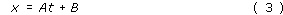where A and B are constants. Different cases of uniform motion simply correspond to different values for the constants A and B. Let us now investigate this equation to see how it conveys information about gradients and intercepts, or equivalently, uniform velocities and initial positions.

To start with, note that according to Equation 3, the position of the particle when t = 0 is just x = B. Thus B represents the initial position of the particle, the intercept of its position-time graph.

In a similar way, note that according to Equation 3, at time t = t﻿1 the position of the particle, let's call it x﻿1, is given by x﻿1 = At1 + B; and at some later time t = t﻿2 the position of the particle is x﻿2 = At﻿2 + B. Now, as we have already seen, the velocity of a uniformly moving particle is defined by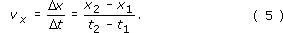so, substituting the expressions for x﻿1 and x﻿2 that we have just obtained from Equation 3 we find that in this particular case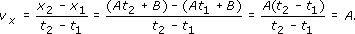Thus, the constant A in Equation 3 represents the particle's velocity, i.e. the gradient of its position-time graph.

We can now read the equation of a straight line, interpreting it graphically or physically. It is

The physical significance of the constants A and B can be emphasised by using the symbols v﻿x﻿ and x0 in their place. Doing this, we obtain the standard form of the uniform motion equations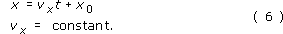So, the uniform motion of the car we have been considering, which has velocity vx = 3.0 m s−1 and initial position x0 = 30 m, can be described by the equation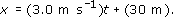This contains just as much information as the graph in Figure 12b.

## Question 11

Write down the equation that describes the uniform motion of the pedestrian who was the subject of Figure 12a.

The equation will have the general form of Equation 6a, with velocity vx = 1 m s﻿−﻿1 (found from the gradient) and initial position x﻿0 = − 20 m (found from the value of x when t = 0). Consequently the required equation may be written: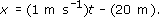A pictorial interpretation of Equation 6a is given in Figure 16.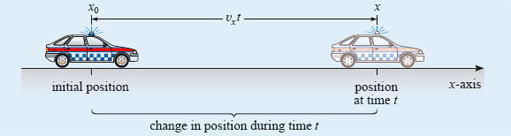Figure 16: A pictorial interpretation of the equation of uniform motion

Equations have the great advantage that they can be rearranged to make them fit the problem at hand. For instance, if you were flying at constant velocity from Paris to Rome, you might well be more interested in your displacement from Paris rather than your position in some arbitrary coordinate system. In mathematical terms, you might be more interested in sx = xx﻿0 rather than x itself. Equation 6a can be rearranged to suit this interest by subtracting x 0 from each side: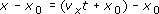and this may be rewritten in the compact form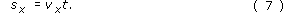Remembering that vx is a constant when the motion is uniform, Equation 7 is equivalent to Equation 6a. It relates the displacement from the initial position directly to the time of flight and thus facilitates working out the distance travelled,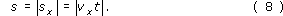The crucial point to remember when manipulating an equation is that both sides of an equation represent the same value. So, if you add or subtract a term on one side of an equation, you must add or subtract an identical term on the other side. The same principle applies to multiplication and division, and to other operations such as taking magnitudes, squaring or taking square roots. (In the latter case you must remember that a positive quantity generally has two square roots; 2 is a square root of 4, but so is −2.) There are two rules to bear in mind when rearranging equations:

1. When one side of an equation consists of several terms added together, each of those terms must be treated in the same way. (It's no good multiplying the first term on one side of an equation by some quantity if you forget to do the same to all the other terms on that side.)

2. When dividing both sides of an equation by some quantity you must ensure that the quantity is not zero. (For instance, it is only legitimate to divide both sides of Equation 7 by xx0if xx0, i.e. if x is not equal to x﻿0.)

The following question is quite straightforward, and there are many ways of answering it, but each will require you to manipulate the uniform motion equations to some extent. As you work out your answer think carefully about each of the procedures you are performing and write it out in words.

## Question 12

When the time on a certain stop-watch is 100 s, a vehicle is positioned at x = −2 m with respect to a certain one-dimensional coordinate system. If the velocity of the vehicle is −12 m s−1, find its position when the stop-watch shows 250 s.

Since vx = constant in this case, the motion is described by the uniform motion equation x = vxt + x0. However, on this occasion we are not given the value of x0, though we are given enough information to work it out. Substituting x = −2 m and t = 100 s into the equation we find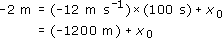Adding 1200 m to both sides shows that 1198 m = x0.

It follows that for this particular motion the equation of uniform motion takes the form x = (−12 m s−1)t + (1198 m).

Consequently, when t = 250 s,

x = −3000 m + 1198 m = − 1802 m.

An alternative method that requires slightly fewer manipulations may be based on the definition of the gradient and the given value of the velocity.

S207_2